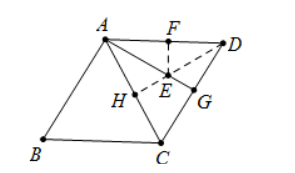【答案】 解 : 如图作 $D H \perp A C$ 垂足为 $H$ 与 $A G$ 交于点 $E$,
$\because$ 四边形 $A B C D$ 是菱形,
$\because A B=A D=C D=B C=6$,
$\because \angle B=60^{\circ}$,
$\therefore \angle A D C=\angle B=60^{\circ}$,
$\therefore \triangle A D C$ 是等边三角形,
$\because A G$ 是中线,
$\therefore \angle G A D=\angle G A C$
$\therefore$ 点 $H$ 关于 $A G$ 的对称点 $F$ 在 $A C$ 上, 此时 $E F+E D$ 最小 $=D H$.

$\therefore C H=\frac{1}{2} D C=3, D H=\sqrt{C D^2-C H^2}=\sqrt{6^2-3^2}=3 \sqrt{3}$,
$\therefore E F+D E$ 的最小值 $=D H=3 \sqrt{3}$